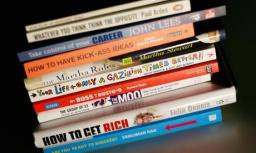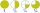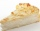# Self

A math text book is 2 2/9 inches thick. how many of these books will fit on a 120-inch self?

Correct result:

n =  54

#### Solution:We would be pleased if you find an error in the word problem, spelling mistakes, or inaccuracies and send it to us. Thank you!Tips to related online calculators
Do you want to calculate greatest common divisor two or more numbers?
Need help calculate sum, simplify or multiply fractions? Try our fraction calculator.
Do you want to convert length units?

## Next similar math problems:

• Fraction and a decimalWrite as a fraction and a decimal. One and two plus three and five hundredths
• Mixed2improperWrite the mixed number as an improper fraction. 166 2/3
• Fraction to decimalWrite the fraction 3/22 as a decimal.
• Evaluate expressionCalculate the value of the expression z/3 - 2 z/9 + 1/6, for z = 2
• ZdeněkZdeněk picked up 15 l of water from a 100-liter full-water barrel. Write a fraction of what part of Zdeněk's water he picked.
• In fractionsAn ant climbs 2/5 of the pole on the first hour and climbs 1/4 of the pole on the next hour. What part of the pole does the ant climb in two hours?
• A quotientWhat is the quotient of 3/10 divided by 2/4 as a fraction
• Cake 71/3 of a cake shared with 4 people. What share of the whole cake has each people?
• Unit rateFind unit rate: 6,840 customers in 45 days
• Pizza 5You have 2/4 of a pizza and you want to share it equally between 2 people how much pizza does each person get?
• Two dividedTwo divided by nine tenths.
• Product of two fractionsProduct of two fractions is 9 3/5 . If one of the fraction is 9 3/7. Find the other fraction.
• Apartment areaAnton wanted to measure the area of the apartment. But he bought a meter only 1.5m long. Later he recalled that he had two meters long at 4.5m and 18m at home. How many times are home gauges longer than the meter he bought?
• Fractions 4How many 2/3s are in 6?
• A bakerA baker has 5 1/4 pies in her shop. She cut the pies in pieces that are each 1/8 of a whole pie. How many pieces of pie does she have?
• Lengths of the poolMiguel swam 6 lengths of the pool. Mat swam 3 times as far as Miguel. Lionel swam 1/3 as far as Miguel. How many lengths did mat swim?
• Tallest and shortestJenn is the tallest on the team. He is 1 1/2 times as tall as the shortest girl whom is 4 1/4 feet tall. How tall is Jebb?